# Assignment 3: Modeling response times

*** Due date: Start of class in Week 4 ***

## Part 1: Tweak the model from last week to implement the threshold crossing decision process (3 points)

• Follow the course material to create a script that keeps track of Δ over time
• Implement a threshold crossing process to make the decision with a while loop
• Plot Δ as a function of time for one decision, remember to label your axes and make your plots look good
• Compute the decision time and accuracy of this one decision

## Part 2: Make a function to implement one decision and have it report the accuracy and decision time (2 points)

• Turn your script into a function that computes the accuracy and decision time. You should be able to call your function like this
[choice, DT] = simulate_ddm(D, f, z);

## Part 3: Plot a histogram of response times (2 points)

• Use your function to simulate 1,000 decisions with a single value of D, f, and z
• Use the hist function to plot a histogram of response times, remember to label your axes
• Compute the average accuacy and average response time for these 1,000 choices

## Part 4: Compute the accuracy and decision time for different values of z (3 points)

• Compute the average accuracy and decision times for a range of z values
z_vals = [2 4 8 16 32];
• Plot the average accuracy as a function of z
• Plot the average decision time as a function of z
• Illustrate the speed accuracy tradeoff by plottin the average accuracy as a function of the average decision time.

## Part 5: Explore the analytic expressions for accuracy and response time (2 extra credit points)

In a slightly more general version of the drift diffusion model, we make things slightly more abstract and say the model is integrating "evidence" for one option or another. We call this evidence y, and at each time point we assume that the evidence changes by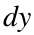which is given by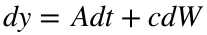where A is the "drift rate" - i.e. the average rate at which evidence comes in in favor of the correct answer -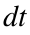is the time step - c determines the standard deviation of the noise and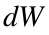is a little piece of random noise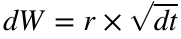where r is sampled from a Gaussian distribution with standard deviation 1 and mean 0.
The decision is made when y crosses a threshold at either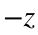(wrong answer) or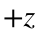(correct answer).
• First, simulate this evidence accumulation process and create a function that computes the accuracy and decision time of one choice that you can call like this ...
[acc, DT] = oneChoice(A, c, z);
• Good parameter values are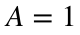,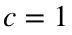and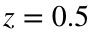• Repeat this process 1,000 times and compute an average accuracy and decision time for your chosen parameter values.
For this model, it turns out we can actually compute analytic expressions for the accuracy and the decision timewhereandand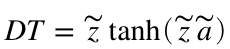• Compute the analytic values for the same set of parameters you used in your simulation. Compare the simulated values with the analytic values. Asgets small they should be almost identical.
Finally, we get use the analytic expressions to figure out an optimal threshold value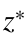that optimally balances speed and accuracy. To do this we need to figure out what we are trying to optimize. One thing we may want to do (and that humans and animals actually appear to do) is to maximize the rate at which we make correct answers. That is the average accuracy divided by the average time to make a decision - the reward rate (RR):where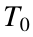denotes a fixed time we can't reduce by changing z - for example, how long it takes to press the button, or how much time passes between trials. For our purposes let's assume thatis 1 second.
• To find the optimal value of z, first compute analytic values for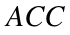and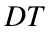as a function of z.
• Then put them into the above equation to get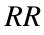as a function of z.
• Then plotvs z. Hopefully you can see there is a particular value of z at which the reward rate is maximized - that is the optimal threshold to balance the speed accuracy tradeoff.